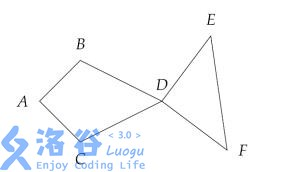# P5286 [HNOI2019]鱼

• 86通过
• 442提交
• 题目提供者 chen_zhe Aya
• 评测方式 云端评测
• 标签 各省省选 2019 湖南
• 难度 NOI/NOI+/CTSC
• 时空限制 2000ms / 512MB
• 提示：收藏到任务计划后，可在首页查看。

## 题目背景

$\text{\color{white}本题数据听说没问题？}$

## 题目描述

在平面坐标系上给定$n$个不同的整点（也即横坐标与纵坐标皆为整数的点）。我们称从这$n$个点中选择6个不同的点所组成的有序六元组$(A,B,C,D,E,F)$是一条“鱼”，当且仅当：$AB=AC,BD=CD,DE=DF$（身形要对称），并且$\angle BAD,\angle BDA$ 与$\angle CAD,\angle CDA$都是锐角（脑袋和屁股显然不能是凹的），$\angle ADE,\angle ADF$ 大于$90^\circ$（也即为钝角或平角，为了使尾巴不至于翘那么别扭）。

下图就是一个合法的鱼的例子：其中点的组成相同，但顺序不同的鱼视为不同的鱼，即$(A,B,C,D,E,F)$和$(A,C,B,D,E,F)$视为不同的两条鱼（毕竟鱼也有背和肚子的两面），同理$(A,B,C,D,E,F)$和$(A,B,C,D,F,E)$也可以视为不同的两条鱼（假设鱼尾巴可以打结）。

问给定的$n$个点可以构成多少条鱼。特别的，数据保证$n$个点互不重复。

## 输入输出格式

输入格式：

第一行一个正整数$n$，代表平面上点的个数。

接下来$n$行每行两个整数$x,y$，代表点的横纵坐标。

输出格式：

输出一行一个非负整数，代表鱼的个数。

## 输入输出样例

输入样例#1： 复制
8
-2 0
-1 0
0 1
0 -1
1 0
2 0
3 1
3 -1
输出样例#1： 复制
16

## 说明

对于前 20%的数据，保证$n \leq 10,|x|,|y| \leq 5$

对于前 40%的数据，保证$n \leq 300$，$0 \leq |x|,|y| \leq 10^5$。

对于另外 20%的数据，保证$|x|,|y| \leq 20$。

对于所有数据，保证$6 \leq n \leq 1000$，$0 \leq |x|, |y| \leq 10^9$，$n$个点互不重复。

提示
标程仅供做题后或实在无思路时参考。
请自觉、自律地使用该功能并请对自己的学习负责。
如果发现恶意抄袭标程，将按照I类违反进行处理。